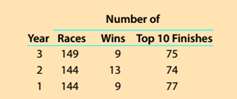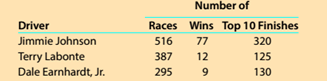Chapter 13, Problem 13.2.3MBA

Chapter
Section
Textbook Problem

Process yield Hendrick Motorsports sponsors cars and drivers in the National Association for Stock Car Auto Racing (NASCAR)'s Sprint Cup Series. Performance data for three recent years are as follows:Performance data for Hendrick Motorsports' drivers are as follows:Using the number of wins as a yield, determine the process yield for each driver. Round to one decimal place.

To determine

Concept Introduction:

Standard Costing System:

Standard Costing system allows estimating the costs, preparing budgets for future periods, and analyzing the performance by comparing the budgets with actual results and find variances.

To Calculate:

The Process Yield for each of the three drivers

Explanation

The Process Yield for each of the three drivers is calculated as follows:

 Driver Races Wins Process Yield A B

Still sussing out bartleby?

Check out a sample textbook solution.

See a sample solution

The Solution to Your Study Problems

Bartleby provides explanations to thousands of textbook problems written by our experts, many with advanced degrees!

Get Started

Find more solutions based on key concepts